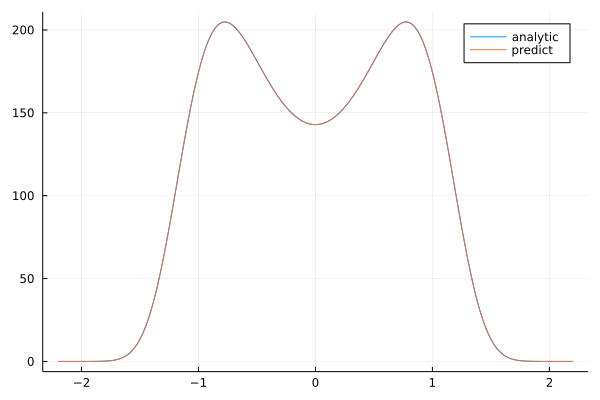# Imposing Constraints on Physics-Informed Neural Network (PINN) Solutions

Let's consider the Fokker-Planck equation:

$$$- \frac{∂}{∂x} \left [ \left( \alpha x - \beta x^3\right) p(x)\right ] + \frac{\sigma^2}{2} \frac{∂^2}{∂x^2} p(x) = 0 \, ,$$$

which must satisfy the normalization condition:

$$$\Delta t \, p(x) = 1$$$

with the boundary conditions:

$$$p(-2.2) = p(2.2) = 0$$$

with Physics-Informed Neural Networks.

using NeuralPDE, Lux, ModelingToolkit, Optimization, OptimizationOptimJL
using Integrals, IntegralsCubature
import ModelingToolkit: Interval, infimum, supremum
@parameters x
@variables p(..)
Dx = Differential(x)
Dxx = Differential(x)^2

α = 0.3
β = 0.5
_σ = 0.5
x_0 = -2.2
x_end = 2.2
# Discretization
dx = 0.01

eq  = Dx((α*x - β*x^3)*p(x)) ~ (_σ^2/2)*Dxx(p(x))

# Initial and boundary conditions
bcs = [p(x_0) ~ 0. ,p(x_end) ~ 0.]

# Space and time domains
domains = [x ∈ Interval(x_0,x_end)]

# Neural network
inn = 18
chain = Lux.Chain(Dense(1,inn,Lux.σ),
Dense(inn,inn,Lux.σ),
Dense(inn,inn,Lux.σ),
Dense(inn,1))

lb = [x_0]
ub = [x_end]
function norm_loss_function(phi,θ,p)
function inner_f(x,θ)
dx*phi(x, θ) .- 1
end
prob = IntegralProblem(inner_f, lb, ub, θ)
norm2 = solve(prob, HCubatureJL(), reltol = 1e-8, abstol = 1e-8, maxiters =10);
abs(norm2)
end

discretization = PhysicsInformedNN(chain,
GridTraining(dx),

@named pdesystem = PDESystem(eq,bcs,domains,[x],[p(x)])
prob = discretize(pdesystem,discretization)
phi = discretization.phi

sym_prob = NeuralPDE.symbolic_discretize(pdesystem, discretization)

pde_inner_loss_functions = sym_prob.loss_functions.pde_loss_functions
bcs_inner_loss_functions = sym_prob.loss_functions.bc_loss_functions
aprox_derivative_loss_functions = sym_prob.loss_functions.bc_loss_functions

cb_ = function (p,l)
println("loss: ", l )
println("pde_losses: ", map(l_ -> l_(p), pde_inner_loss_functions))
println("bcs_losses: ", map(l_ -> l_(p), bcs_inner_loss_functions))
return false
end

res = Optimization.solve(prob,LBFGS(),callback = cb_,maxiters=400)
prob = remake(prob,u0=res.u)
res = Optimization.solve(prob,BFGS(),callback = cb_,maxiters=2000)

And some analysis:

using Plots
C = 142.88418699042 #fitting param
analytic_sol_func(x) = C*exp((1/(2*_σ^2))*(2*α*x^2 - β*x^4))

xs = [infimum(d.domain):dx:supremum(d.domain) for d in domains]
u_real  = [analytic_sol_func(x) for x in xs]
u_predict  = [first(phi(x,res.u)) for x in xs]

plot(xs ,u_real, label = "analytic")
plot!(xs ,u_predict, label = "predict")